Courses

# Work and Energy, Chapter Notes, Class 9, Science Class 9 Notes | EduRev

Created by: Learners Habitat

## Class 9 : Work and Energy, Chapter Notes, Class 9, Science Class 9 Notes | EduRev

The document Work and Energy, Chapter Notes, Class 9, Science Class 9 Notes | EduRev is a part of Class 9 category.
All you need of Class 9 at this link: Class 9

Work and Energy

WORK

The intuitive meaning of work is quite different from the scientific definition of work. In everyday activity, the term 'work' is used equally for mental work and for physical work (involving muscular force) as is clear from the following examples.

(i) You may read a book or exert yourself mentally in thinking about a simple or difficult problem.

(ii) You might be holding a weight without moving.

(iii) You may be carrying a load and moving with uniform velocity.

(iv) You may be trying hard to move a huge rock which does not move despite your best efforts, though you may get completely exhausted in the process.

In all these cases, according to scientific definition, you are not doing any work.

Scientific conception of work

In physics, the term work is used in a special technical sense and has a much more precise definition which follows from the following examples.

(i) When a box is pushed on a floor by applying a force and it moves through some distance, work is said to be done. In this case, the applied force displaces the box.

(ii) When we pull a trolley by applying a force and it moves through some distance, work is again said to be done.

(iii) When we lift a box through a height, we have to apply force. In this case, the applied force does work in lifting the box.

From all the examples given above, it follows that work is done if :

• a force is applied on the object and
• the object is displaced from its original position.

No work is said to be done if any of the two conditions is not satisfied.

Work: When Constant Force is Acting in the Direction of Displacement

A body A is kept on a smooth horizontal surface. A force F is applied as shown. This force acts on the body for some time during which the displacement of the body is s. In such a case work is defined as follows.

Work done by a force on a body is the product of force and displacement of the body in the direction of the force.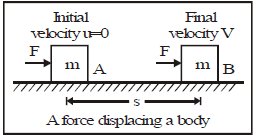Work = Force × Displacement

⇒ W = F × s

Work is a scalar quantity. This means that it has no sense of direction.

Unit of Work

In cgs system unit of work = erg

1 erg = If a force of one dyne displaced a body through a distance of one centimetre then work is 1 erg

1erg = 1 dyne × 1 cm

In SI unit of work is = joule(J)

When a force of 1N acts on an object and the object moves a distance of 1m in the direction of the force, the work done by the force is 1J.

______________________

Definition of one Joule

When F = 1N, s = 1m, then W = 1J

If a displacement of 1m is produced by a force of 1N acting in the direction of displacement then the work done by the force is 1J.

1J= 1 N x 1 m = 105 dyne × 102 cm =107 erg

______________________

Conclusion

1. When s = 0, W = 0, i.e. work done by a force on a

body is zero if the displacement of the body is zero. For example, when you push a wall with a force F, then the displacement of the wall is zero. Therefore, the work done by force F on the wall is zero.

2. When you sit on a chair and prepare a lesson in two hours, you may feel tired. But according to physics, no work is done.

3. If you hold a briefcase for one hour and do not move the briefcase, then s = 0. Therefore, work done by you on the briefcase is zero.

Work: When a Constant Force is Acting at an Angle to the Displacement

Let us consider a body A lying on a smooth horizontal surface. A constant force F acts at an angle q to the horizontal. The body is displaced through distance in the horizontal direction. Here the complete force F is not responsible to displace the body. A part of the force acting in the direction of displacement is responsible for displacing the body. This horizontal part is F cos q. Work done in this case is defined as 'work done by a constant force acting at an angle to the displacement is the product of component of force in the direction of displacement and the displacement of the body'.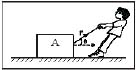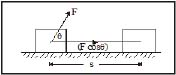Work = Component of force in the direction of displacement x Displacement

W = (F cos θ ) × s

W = F s cos θWork done is maximum when force acts in the direction of displacement.

Case-1 : Work done is positive when q is acute. This is because cos q is positive, Work done is maximum when θ = 0°. This is because the maximum value of cos θ = 1. This happens when force acts in the direction of displacement.

Case-2 : When the angle between force and displacement is 90°, i.e. when θ = 90°, then

cos θ = 0 cos 90° = 0

W= 0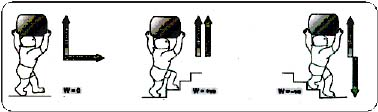Some examples where work done is zero because θ = 90°

(a) Work done by centripetal force

When a stone is whirled in a horizontal circle, then centripetal force acts at 90° to the displacement. Therefore, work done by centripetal force is zero.

(b) Work done by coolie

When a coolie moves on a horizontal surface, he applies a force on the load kept on his head in vertically upward direction. Therefore, θ = 90°. Therefore, work done by the force applied by coolie is zero.

(c) Motion of the Earth around the Sun

Work done by the centripetal force (which is the gravitational pull of sun on earth) acting on earth is zero. Because centripetal force is perpendicular to the displacement.

[Remember the displacement is always tangential and centripetal force is always radially inward. The angle between radius and tangent is 90°].

Case-3 : When angle between force and displacement is 180°, i.e. when q =180° then cos 180° = _1.

In this case work done is negative.

Let us take an example where work done is negative because q = 180°

A block A is pushed by the force F and displaced through s. Work done by applied force,

W = F × s × cos 0°

= Fs

Work done by frictional force,

W = f × s × cos 180°

= _fs

MEMORlSE : Work may be positive, negative or zero depending on the angle

ENERGY

When a car runs, the engine of the car generates a force which displaces the car. In other words, work is done by the car. This work is done on the expense of fuel. Fuel provides the energy needed to run the car. Had the petrol tank been empty, car could not be run. The conclusion is that, if there is no source of energy, no work will be done.

Let us take another example. Suppose a lift takes some persons from ground floor to second floor. Then the lift performs work. If you enquire, you will find that the lift is operated by an electrical motor. Thus, electrical energy does the work. If there is no electricity the lift will not operate. Again if there is no source of energy, no work will be done.

The above statement is not just true for the above two examples, but is true for all processes.

Therefore, energy is defined as the capacity to do work.

More the energy in a body, more is the work the body can do.

Energy is a scalar quantity.

The S.I. unit of energy is joule (J). Bigger units is 1 kJ = 1000 J, 1 MJ = 106 J

The C.G.S. unit of energy is erg.

 MEMORISE : Energy is the ability to do work. More the energy, more the work that can be performed and vice-versa.

Forms of energy

Nature has been very kind to us in providing us energy in various forms. These forms of energy are as follows.

1. Solar energy. The energy radiated by the Sun is called solar energy. Plants collect and store this energy to make food through photosynthesis.

2. Heat energy. It is the energy released when coal, oil, gas or wood burn and it produces in us the sensation of warmth.

3. Light energy. It is the form of energy which produces in us the sensation of light. Sun is the natural source of light.

4. Chemical energy. It is the energy possessed by fossil fuels (coal; petroleum and natural gas) and is also called the fuel energy. The food that we eat has chemical energy stored in it.

5. Hydro energy. The energy possessed by water flowing in rivers and streams is called hydro energy. This energy is used to generate electricity in hydroelectric power plants.

6. Wind energy. The energy possessed by moving air is called wind energy.

7. Ocean thermal energy (OTE). Solar energy stored in the oceans in the form of heat is called ocean thermal energy.

8. Geothermal energy. It is the heat energy of the Earth and is found within rock formations and the fluids held within those formations.

9. Biomass energy. It is the energy obtained from biomass (i.e., living matter or its residues).

10. Tidal energy. It is the energy derived from the rising and falling ocean tides.

11. Sound energy. It is the energy possessed by vibrating objects and it produces in us the sensation of hearing.

12. Mechanical energy. It is the energy possessed by a body due to its position (or configuration) or motion. The energy possessed due to position or configuration is called potential energy and that due to motion is called kinetic energy. The sum of these two energies is called the mechanical energy.

13. Electric energy. The energy possessed by charges (either at rest or in motion) is called electric energy.

14. Magnetic energy. It is the energy possessed by magnetised bodies e.g. a magnet.

15. Electromagnetic energy. It is the general name for electric and magnetic energies.

16. Nuclear energy. The energy produced in the processes of fission and fusion is called nuclear energy.

KINETIC ENERGY

A moving object is capable of doing work because of it" motion. Hence, we say that the object has kinetic energy. "Kinetikos" in Greek means "to move". Hence,kinetic energy means energy due to motion. The energy is stored in the object when work is done to change its velocity from a lower value to a higher value, or from rest to certain velocity.

Kinetic energy of an object is defined as the energy which it possesses by virtue of its motion, and is measured by the amount of work that the object can do against an opposing force before it comes to rest.

Kinetic energy of an object moving with a certain velocity is equal to the work done on it to enable it to acquire that velocity.

Examples of bodies possessing kinetic energy

1. A ball rolling on a surface because it can set another ball into motion by striking it.

2. A bullet fired from a gun as it is able to penetrate some distance into a target which it strikes.

3. A tarpedo in motion as it can do work by penetrating into the side' of a ship.

4. Water in motion as it can turn a wheel or a turbine.

5. A fast wind as it can set a boat in motion when striking against its sail.

6. A moving hammer as it drives a nail into a wall against the resistance offered to it by the wall.

7. A falling body as it can break something on which it falls.

Expression for Kinetic Energy

Consider an object of mass m which is moving with an initial velocity u on a perfectly frictionless surface. Let a constant external force F act on it and produce an acceleration a in it. If v is the final velocity of the object after having undergone a displacements, then from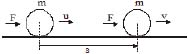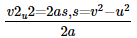.........(1)

Work done by the force in displacing the body through s, i.e.,

W= F × s ..........(2)

We know from Newton's Second Law of Motion,

F = ma ...........(3)

From equation (1), (2) and (3), we get

W = (ma) ×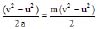or W =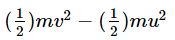......... (4)

If the object is initially at rest, u = 0 and as such from eqn. (4),

W =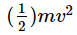...........(5)

This work done (W) in making the object acquire a velocity v after starting from rest has not gone waste and is, in fact, stored in the object.

Work stored up in a moving object is called the kinetic energy of the object.

If kinetic energy of an object is denoted by Ek then

Ek =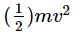..............(6)

Kinetic energy of a moving object is defined as half the product of the mass of the object and the square of the speed of the object.

POTENTIAL ENERGY

The energy possessed by an object by virtue of its position or configuration is called its potential energy. It is measured by the work that the object can do in passing from its present position or configuration to some standard position or configuration (known as zero position or zero configuration)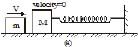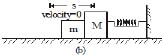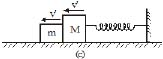The kinetic energy of mass m converts into elastic potential energy of spring.

Let us take an example :- Let a small mass m be released from a smooth inclined plane. Another mass M is kept at a rough horizontal plane at rest. The mass m will move along the inclined plane and strike the mass M. Both the masses will move along the horizontal surface for some distance and come to rest. The mass M moves a distance s by the force applied by m. Thus, m does work for which it requires energy. This energy is possessed by m at A as it was at a height h from the horizontal surface. This energy due to position is called potential energy. Precisely speaking this energy is called gravitational potential energy.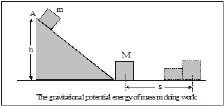Thus potential energy is defined as follows :

The energy possessed by a body due to its position or change in shape is called potential energy.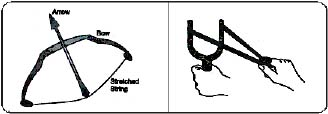Note : The energy possessed by a body due to its height from the surface of earth is called gravitational potential energy and that due to change in shape is called elastic potential energy.

Other examples where elastic potential energy is stored are :

(i) a stretched bow

(ii) a stretched rubber band

(iii) a wound spring

All above examples are because of change in shape.

 Expression for Gravitational Potential Energy Let us consider a block of mass m kept on the surface of earth. Let the block be lifted to a height h. For that a force F is required which is equal to mg. This force lifts the block through a distance h. The work done by this force,

W = F × h

∴  W = mg × h [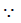F = mg]

This work is converted into potential energy (P.E.) of the block.

P.E = mgh

The expression shows that potential energy depends on

(a) mass m

(b) height h from ground

(c) acceleration due to gravity g

TRANSFORMATION OF ENERGY

We have discussed various forms of energy available to us. We convert energy from one form to another. Given by following examples.

1. Conversion of mechanical energy into electrical energy. The potential energy of water stored in a dam is changed to kinetic energy when it falls from a height. This kinetic energy rotates a turbine to produce electric energy.

2. Conversion of electrical energy into mechanical energy. An electric motor uses electrical energy to run various electrical appliances, e.g., a train, a fan, washing machine, mixer, grinder etc.

3. Conversion of electrical energy into heat energy. In an electric heater, a geyser, a toaster, an oven etc., electric energy is changed to heat energy.

4. Conversion of heat energy into mechanical energy. In heat engines (e.g., a steam engine), heat energy changes to mechanical energy.

5. Conversion of electrical energy into light energy. In an electric bulb, a fluorescent tube, a flood light etc., electrical energy is changed to light energy.

6. Conversion of electric energy into sound energy. An electric bell, a stereo, a loudspeaker etc., change electric energy into sound energy.

7. Conversion of chemical energy into heat energy. When fuels are burnt, chemical energy gets converted into heat energy.

8. Conversion of electrical energy into chemical energy. When a battery is charged, electrical energy changes into chemical energy. An inverter in our home does the same thing.

9. Conversion of sound energy to electrical energy. A microphone converts sound energy into electrical energy.

10. Conversion of chemical energy to electrical energy. An electric cell converts chemical energy into electrical energy.

11. Conversion of light energy into electric energy. A solar cell converts light energy into electrical energy.

12. Conversion of chemical energy into mechanical energy. In automobiles, chemical energy of petrol, diesel or CNG (compressed natural gas) is converted into mechanical energy.

13. Conversion of light energy into chemical energy. In photosynthesis, light energy .from the Sun is absorbed by green plants and is converted to chemical energy.

14. Conversion of nuclear energy into electrical energy. Nuclear power. plants are used to generate electrical energy from nuclear energy.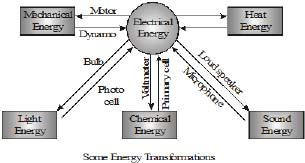Some man made devices which convert one form of energy into another are is given as follows.

 Sr. Device Input energy Output energy 1. Fan Electrical energy Kinetic energy 2. Electric lamp Electrical energy Light energy 3. Electrical heaters Electrical energy Heat energy 4. Radio Electrical energy Sound energy 5. Water pump Electrical energy to kinetic energy of impeller to potential energy of water 6. Cell Chemical energy Electrical energy ​7. Microphone Sound energy Electrical energy 8. Rechargeable cell (a) During discharging  Chemical energy (b) During charging Electrical energy (a) Electrical energy (b) Chemical energy ​9. Loudspeaker Electrical energy Sound energy 10. Elevator moving up Electrical energy Potential energy 11. Television Electrical energy Sound energy, light energy 12. Thermal power plant Chemical energy of coal Electrical energy 13. Car Chemical energy of petrol/diesel Mechanical energy ​14. Nuclear power plant Nuclear energy Electrical energy 15. Solar cell Solar energy Electrical energy 16. Watch Potential energy of wound spring K.E. of hands of watch 17. Generator Kinetic energy Electrical energy

Law of conservation of energy

According to law of conservation of energy, energy can neither be created nor destroyed, it can be converted from one form to another.

CONSERVATION OF MECHANICAL ENERGY

Let us consider two cases where mechanical energy is conserved.

Case : A ball is dropped from some height.

At point A: Let a ball of mass m is dropped from a height h. Here the total energy (T.E.) of the ball is the sum of kinetic energy (K.E.) and potential energy (P.E.).

Potential energy = mgh

Kinetic energy = 1/2 m (0)2 = 0 (u = 0)

∴  [T.E.]A = mgh 0 = mgh ... (A)

At point B: Let the ball travel a distance of h1 in time t during its fall. Then the velocity of the ball after time t can be found by using equation of motion. u = 0, a = g, S = h1, v = v

Using v2 - u2 = 2as

v2 - u2 = 2gh1

Þ v2 = 2gh1

Now K.E. at B =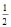mv2 =m × 2gh1 = mgh1

P.E. at B = mg (h - h1)

Total energy at B = K.E. P.E. = mgh1  mg(h - h1) = mgh ... (B)

At point C : Suppose the ball cover a distance h when it moves from A to C. Let V be the velocity of the ball at point C just before it thouches the ground, then

v2 u2 = 2gh

v2 0 = 2gh or v2 = 2gh therefore

Kinetic energy (K.E.) = 1/2 mv2 = 1/2 m (2gh) = mgh

and potential energy at C P.E. = 0

Hence total energy at point C.

E = K.E. P.E. = mgh 0 = mgh ... (C)

Thus it is clear from equations A, B and C, that the total mechanical energy of a freely falling ball remain constant. There is, simply, a transformation of mechanical energy. This transformation is depicted in the graph of figure.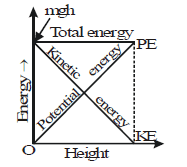POWER

1. Power of a machine is defined as the rate of work done by the machine.

2. Power is defined as the rate of doing work.

3. Power of a machine is defined as work done by the machine per second.

If you lift a block of mass 1 kg through a distance of 1 m in 2 seconds, what is the work done?

W = F × s = = mg × h = 1 × 9.8 × 1 = 9.8 J

If you lift the same block through the same height in 1 minute, what is the work done? The answer comes out to be the same 9.8 J.

What is the work done if the time taken is 5 minutes? The work done is again 9.8 J.

But we are generally interested in time oriented work, i.e., work should be completed in a particular amount of time. The physical quantity which takes care of 'how fast is the work done' is power.

Note: If we want to find rate of work done by a man then the word 'machine' can be replaced by 'man'.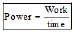or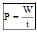Power is scalar quantity

Unit of power

SI unit of power is watt (W), 1 kW = 1000 W, 1 MW (Mega watt) = 106 W

Another unit of power is horse power (HP). 1GW(giga watt) = 109 W

1 HP = 746W

Definition of Watt

When t = 1 s, W = 1J, then P = 1 W

If the work done by a machine is 1 joule in a time of 1 second, then the power of the machine is 1 watt.

Power in terms of energy :

Since work and energy are interconvertible, therefore,

Power =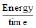or P =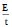E = P × t

Also, W = F × s When displacement is applied in the direction of force

then P =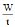= F ×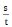Þ P = F × v This is power in terms of force and velocity.

Average power : Average power of an agent is defined as the ratio of total work done to the total time taken.

Average Power =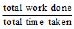Commercial Unit of Energy : Kilowatt-hour (kwh)

One kilowatt hour is the amount of energy consumed (or work done) by an agent in one hour working at a constant rate of one kilowatt.

Is kWh a unit of power or energy?

We can write 1 kWh as 1 kW × 1h.

Now, since P =∴ E = P × t

If power is in kW (kilowatt) and time in hour, then the unit of energy is kWh.

The unit kWh is important because this is a commercial unit of energy used by electricity boards. If you enquire from your parents what was the last electricity bill? If the answer is 600 units, it means that you have used

600 kWh of energy during the duration of bill. Thus, you pay for the electrical energy that you use.

Relation between kWh and Joule

1 kWh = 1000 Wh [Q 1 kW = 1000 W]

Now, 1 W = 1 Js-1 and 1 h = 60 × 60 s = 3600 s

1kWh = 1000 Js-1 × 3600 s = 3600000 J = 3.6 × 106 J

1 kWh = 3.6 × 106 J = 3.6 MJ

Note : An energy of 1 kWh is equivalent to using a bulb of 1 kW for 1 hour.

_____________________________

Competitive Window:

What can you conclude from the relationship, K.E. =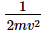we can conclude as follows :

When the velocity of a body is kept constant, the kinetic energy is directly proportional to the mass of the body, K.E.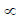m

Thus,

If the mass of a body is doubled (v remaining constant), the kinetic energy of the body also gets doubled.

If the mass of the body is reduced to half (v remaining constant), the kinetic energy of the body also gets halved.

The kinetic energy of a body is directly proprotional to the square of its velocity (or speed) i.e., K.E.v2

So, (Q m is constant)

If the velocity of a body is doubled, then its kinetic energy increases four times.

If the velocity of a body is reduced to half, then its kinetic energy gets to one-fourth.

How is the kinetic energy of a body related to its momentum

Let us consider a body of mass m having a velocity v. Then

Momentum of the body p = Mass × velocity = m × v

This gives, v =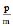... (1)

From definition,

Kinetic energy (K.E.) of the body =... (2)

Substituting the value of v from Equation (1) into Equation (2) we can write,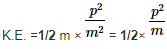Then, we can write, p2 = 2m × K.E.

p =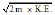Thus, Momentum =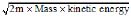,

,

,

,

,

,

,

,

,

,

,

,

,

,

,

,

,

,

,

,

,

,

,

,

,

,

,

,

,

,

;